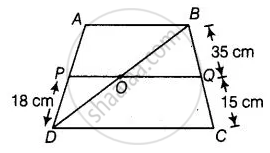# ABCD is a trapezium in which AB || DC and P and Q are points on AD and BC, respectively such that PQ || DC. If PD = 18 cm, BQ = 35 cm and QC = 15 cm, find AD. - Mathematics

Sum

ABCD is a trapezium in which AB || DC and P and Q are points on AD and BC, respectively such that PQ || DC. If PD = 18 cm, BQ = 35 cm and QC = 15 cm, find AD.

#### Solution

Given, a trapezium ABCD in which AB || DC.

P and Q are points on AD and BC, respectively such that PQ || DC. Thus, AB || PQ || DC.Join BD.

In ΔABD,

PO || AB   ......[∵ PQ || AB]

By basic proportionality theorem,

(DP)/(AP) = (DO)/(OB)   .......(i)

In ΔBDC,

OQ || DC    ......[∵ PQ || DC]

By basic proportionality theorem,

(BQ)/(QC) = (OB)/(OD)

⇒ (QC)/(BQ) = (OD)/(OB)   ......(ii)

From equations (i) and (ii)

(DP)/(AP) = (QC)/(BQ)

⇒ 18/(AP) = 15/35

⇒ AP = (18 xx 35)/15 = 42

∴ AD = AP + DP

= 42 + 18

= 60 cm

Concept: Basic Proportionality Theorem (Thales Theorem)
Is there an error in this question or solution?

#### APPEARS IN

NCERT Mathematics Exemplar Class 10
Chapter 6 Triangles
Exercise 6.3 | Q 9 | Page 68

Share